# 13 – First Contact – U(1)

 < ρℝεν | ℂσητεητs | ℕεχτ >“This is the beginning of the journey to complete the unitary system.” – Charles Ross

All the way back in the Introduction we spoke about the particles in the Standard Model being governed by the composite Group denoted by:

SU(3) × SU(2) × U(1)

Towards the end of Chapter 10, we learnt what the ×s in the above mean. The next two Chapters will concentrate on two of the three Groups in question. In this chapter, the focus will be on the least complex of the three, U(1). In the following one, we will look at U(1)’s big brother, U(2), and then explore its Subgroup, SU(2). Our final Group is SU(3). This will be defined in generic terms in the next Chapter, but we will only get into the details of SU(3) once we have introduced some further Mathematical apparatus in Chapter 15.

In earlier chapters of this book, we defined the concept of a Group and introduced two extensions of the numbers that we use day-to-day: matrices and the Complex Numbers. In this Chapter we are first going to extend a Group we met in Chapter 11 to create U(1). We will then go on to use both matrices and Complex Numbers to define a generic Unitary Group. In the next Chapter we will build on this work to construct a specific Unitary Group, U(2), and then show how SU(2) fits into this.

However, prior to leaping in, let me also post a warning. Before we can reach our eventual goal of exploring how Unitary Groups (and their Special kindred, who we will meet later) apply to sub-atomic Physics we will have to pause again in order introduce a few more concepts; so the end of our journey is not yet within sight. With that said, let’s at least sketch out what our destination looks like for U(1). To do this we need to define some new Mathematical structures, but let’s start by looking more closely at one we constructed in an earlier part of this book.

To Infinity and Beyond…Towards the end of Chapter 11, we saw that all of the nth roots of unity can be expressed as powers of any single nth root of unity that is not equal to 1. Most simply, if we take the first such root as we travel anticlockwise from the right-hand x-axis, let’s call it z, then these powers fall in sequence as shown below: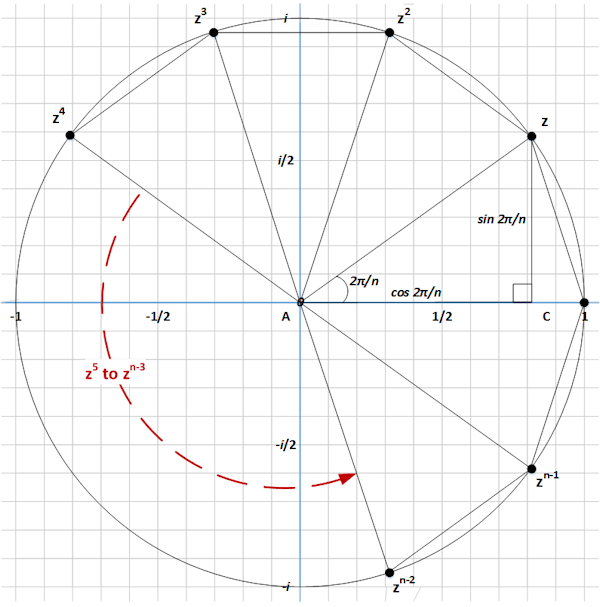Further we saw that {1, z, z2, … , zn-2, zn-1} forms a Group isomorphic to ℤn.

Let’s try to extend this idea by considering not just the specific n complex numbers sitting on a circle of unit radius, but all such complex numbers. Of course this means moving from a finite set, of size n, to an infinite one. During this journey we may begin to spot some familiar landmarks, ones that dotted the countryside during the latter part of Chapter 6. This is not coincidental and we will also see some similarities between our destination in this Chapter and the one we previously reached in Chapter 6.

So let’s be brave and attempt the journey to infinity and beyond. To start this, let’s consider two complex numbers α and β both on the unit circle as below :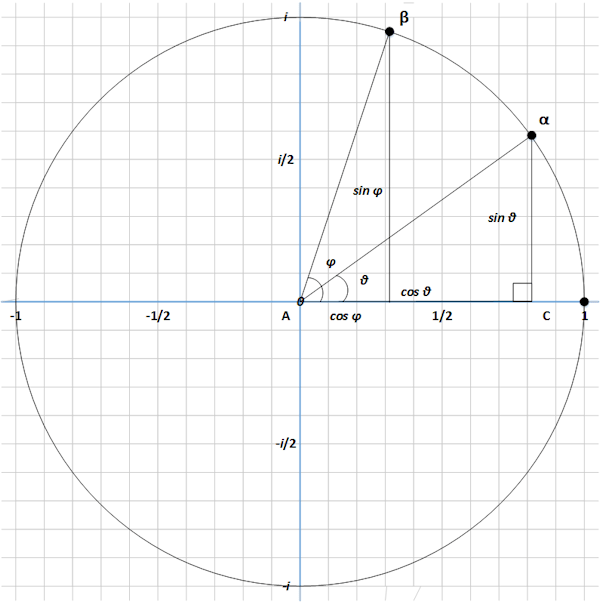Here the angle that α makes with the x-axis is labelled θ and the angle that β makes is labelled φ. As in both Chapter 6 and Chapter 11, basic Trigonometry means that:

α = cos θ + i sin θ

and

β = cos φ + i sin φ

From Euler’s formula, we can also see that:

cos θ + i sin θ = eiθ

and

cos φ + i sin φ = eiφ

Once more relying on basic results for powers, we know that anam = an + m. Applying this to the above we can see that:

eiθ eiφ = ei(θ + φ)

Using Euler’s Formula to move back from exponential  to Trigonometric functions once more we have:

(cos θ + i sin θ)(cos φ + i sin φ) = cos(θ + φ) + i sin(θ + φ)

So if we multiply α and β we get a complex number that is still on the unit circle, but now at an angle of θ + φ .

Previously when we squared an nth root of unity, we got another nth root of unity (by the definition we proved in Chapter 11, this is on the unit circle). But more generally if we multiply any two numbers on the unit circle, we get another number on the unit circle, but one where its angle with respect to the x-axis is given by adding the angles of the two numbers we multiplied. The reader should now begin to be attuned to such things, have we found another Group?

1. Closure

This is what we have just shown above, if we multiply any two Complex Numbers on the unit circle, we get another Complex Number on the unit circle.

2. Identity

It is trivial to see that:

1 × a + ib = a + ib

for all Complex Numbers, including those on the unit circle (and 1 is clearly on the unit circle as well).

3. Inverse

Well if we have established that multiplication is the same as addition of angles, then to get back to the Identity from a point with angle θ, we need to multiply by a point with an angle of precisely -θ, remembering that θ is measured with respect to the x-axis.

If we go all the way back to Chapter 7, we found a way to reflect a Complex Number in the x-axis, which would be equivalent to turning θ into -θ. This is taking the Complex Conjugate. So for our point α defined above as cos θ + i sin θ, its Complex Conjugate is derived by changing the sign of just the Imaginary component and so is given by: cos θ – i sin θ. Using a bar to denote the Complex Conjugate, we then have:

α α = (cos θ + i sin θ)(cos θ – i sin θ) =

(cos θ)2 – (i sin θ)2

Noting that we generally write things like (cos θ)2 as cos2 θ and of course that, as always, i = -1, we get:

cos2 θ + sin2 θ = 1

This last line is a well known identity in Trigonometry and – if you take a look at the diagram above – follows from Pythagoras’s Theorem, where the hypotenuse is the radius of the unit circle and cos θ and sin θ are the coordinates of any point on it (and thus the lengths of the other two sides of a right-angled triangle).

So the inverse of a Complex Number on the unit circle is given by taking its Complex Conjugate .

4. Associativity

As we have transformed multiplication of Complex Numbers on the unit circle to addition of their angles with respect to the x-axis, we have also shown associativity as addition is associative (we have also shown Commutativity).

So all of the (infinite number of) Complex Numbers on the unit circle form a Group under the binary operation of complex multiplication .

The Group we have defined is known as the Circle Group and is denoted by T  so we have:

T = {a + ib such that a, b ∈ ℝ and a2 + b2 = 1}

And there we have it, T is U(1)!

Well actually T is U(1), but the linkage rather breaks down for U(2), U(3) etc. We need to consider these Unitary Groups of larger order because they contain SU(2) and SU(3) as Subgroups and we need SU(2) and SU(3) to properly cover the Standard Model. So let’s make the effort and consider the more general case.

A generic Unitary Group, U(n) [of which U(1) is the simplest example], is defined in slightly different terms to the ones we have been using. Where n is greater than one, this shift in definition will require us bringing together the concepts of matrices and Complex Numbers. The result will be very similar , we will generate Groups which relate to moving through angles round a circle (or analogues of this) and in particular ones where if we combine two elements, it is the same as adding the angles . However in order to focus more precisely on generic Unitary Groups let’s change the playing field from multiplications of individual Complex Numbers to multiplications of Complex Matrices.

Grandes ComplicationsTo start with a comment that is probably not that surprising. When looking at U(n) we are going to consider n × n matrices. To date the matrices we have looked at have had entries in ℤ, ℚ or ℝ. We are now going to consider Complex Matrices, ones whose entries are in ℂ. So if A is a generic 2 × 2 Complex Matrix, it would look something like: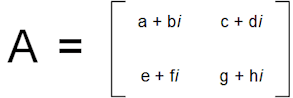Where a, b, …, h ∈ ℝ.

It can be seen that we quickly run out of letters as the matrices get bigger, so to avoid this issue we typically use letters and subscripts, the subscripts relating to the location of an entry in the matrix. Thus if B is a generic 3 × 3 Complex Matrix, it might be denoted by:Where aij and bij ∈ ℝ.

The reader may be glad to know that I won’t be looking at any matrices bigger than 3 × 3, but we will need this size of matrix when we define the larger of our two Special Unitary Groups, SU(3).

At the beginning of this Chapter, I mentioned that we needed to revist a concept introduced in Chapter 6 and to introduce a new one. The first of this is the transpose of a matrix (any matrix, not necessarily a complex one). This is generated by reflecting the entries across the diagonal running from top left to bottom right. So the transpose of our 2 × 2 Complex Matrix, A, which is denoted by AT would be:And that of our 3 × 3 matrix, B, would be: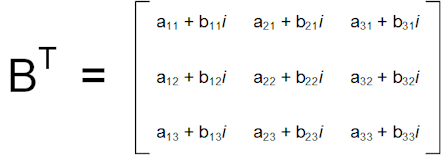In particular looking at the 3 × 3 example, two things are apparent. First the entries on the top left to bottom right diagonal are left unchanged by transposition. Second a generic entry aij + ibij gets moved to where entry aji + ibji used to be and vice versa. The diagonal elements are all of the form aii + ibii where their rows and columns are equal. This is why their position is preserved by transposition.

While transposition applies to any matrix, Complex Matrices have another transformation that they can be subjected to. This is conjugation, which is related to the same concept pertaining to Complex Numbers, something that we introduced towards the end of Chapter 7. Conjugation involves changing the sign of just the Imaginary part of a Complex Number.

So for our 2 × 2 example, A, the conjugate, denoted by A, is given by:And for our 3 × 3 example, B, we have B as follows:Finally we can combine the two of these operations to create the Conjugate Transpose. This consists of two steps. First create the transpose matrix as described above. Second replace each entry with its Complex Conjugate .

The typography for the resulting element is not entirely well-defined and also shifts according to the discipline using the construct. I am going to stick to the Conjugate Transpose of a matrix A being shown as AH .

So for our 2 × 2 example we have: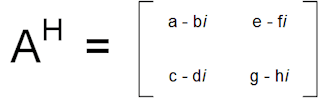And for our 3 × 3 example we have: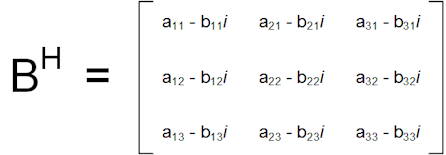Having developed all of this apparatus, we can now offer a definition:

A Unitary Matrix is a matrix M such that its Conjugate Transpose is its inverse. That is:

MMH = MHM = 1 

A second definition follows on from this:

The Unitary Group of degree n, denoted by U(n), is the set of all n × n Unitary Matrices under matrix multiplication.

1 × 1 is Complex…

If we consider the case where n=1, then 1 × 1 matrices are just the Complex Numbers and Matrix Multiplication is just regular multiplication of Complex Numbers. For a 1 × 1 “matrix” transposition is irrelevant (there is nothing off of the diagonal to transpose) and for any Complex Number a + ib, its conjugate is a – ib. Given that the inverse of a + ib is 1/(a + ib), we are looking for all Complex Numbers such that:

a – ib = 1/(a + ib)

Cross multiplying we get:

(a – ib)(a + ib) = 1

Which means that:

a2 – (ib)2 = 1

Recalling that i2 = -1, this becomes:

a2 + b2 = 1

Which is precisely our definition of all Complex Numbers appearing on the unit circle.

So we can define U(1) as follows:

U(1) = {a + ib such that a, b ∈ ℝ and a2 + b2 = 1}

This is the definition we arrived at from our more Geometrically-centred approach in the first instance.

 U(1), SO(2) and Isomorphism In Chapter 6, we examined rotations and reflections enacted by n × n matrices with entries in the Real Numbers. As part of this we met the Special Orthogonal Groups of degree n, SO(n). These exclude reflections and include just rotations in n-dimensional Real spaces. As part of this, we spent some time on the 2 × 2 case, SO(2). Back then, I mentioned that we would later come across another Group isomorphic to SO(2) and we have just introduced this. T, U(1) and SO(2) are all essentially the same Mathematical structure. Because U(1) is based on rotations of the Complex Plane and SO(2) is based on rotations of the 2D Real Plane, it is necessary to create a mapping between the two. If we take the exponential formulation of U(1) that we used to introduce the Group and compare this to Real 2 × 2 matrices in SO(2) our mapping looks like the following:Where I have previously informally used the term isomorphic to explain that two Groups have the same shape, what this really means is that there exists just such a mapping from all the elements of one Group to all the elements of the other Group (and vice versa). More specifically, if our two Groups are G and H and if our mapping from G to H is denoted by ψ then this mapping must have the following properties: For all h ∈ H there exists a unique g ∈ G such that: ψ(g) = h   For all g ∈ G there exists a unique h ∈ H such that: ψ-1(h) = g Here ψ-1 is the inverse of the mapping, so if ψ: G ↦ H, g ↦ h then: ψ-1: H ↦ G, h ↦ g Where the g and h in each mapping are the same Group elements. I.e. ψ-1(ψ(g)) = g and ψ(ψ-1(h)) = h.   If the binary operator on G is ○ and that on H is ◊, then for any g1, g2 ∈ G we must have: ψ(g1 ○ g2) = ψ(g1) ◊ ψ(g2) I.e. the mapping preserves the essence of how Group elements are combined. If a mapping ψ satisfies all of the above, it is called an isomorphism and G and H are said to be isomorphic Groups, ones with the same shape. When this happens, we write: G ≅ H

Having spent some time looking at the 1 × 1 case, it is fair to say that things get somewhat more complicated when we instead move on to 2 × 2. It is to this second Unitary Group, U(2), that I will turn my attention in the next Chapter, this will also focus on a particular Subgroup of U(2), namely SU(2).

 Concepts Introduced in this Chapter Generalisation of Roots of Unity An extension of the concept of the n nth roots of unity, all of which lie on the unit circle, to all Complex Numbers lying in the unit circle. This takes us from a finite to an infinite set and from a discrete Group to a Continuous one. The Circle Group The Group formed by the above generalisation, where the binary operator is multiplication of Complex Numbers. This is isomorphic to the Unitary Group of degree 1, or U(1). Higher order Unitary Groups are defined quite differently and the relationship begins to break down. Conjugate of a Matrix The matrix formed by taking the complex conjugate of all entries, which of course assumes such entries are Complex Numbers. Conjugate Transpose (or Hermitian) A transformation of an n × n Complex Matrix which consists of a transposition (see Chapter 6) followed by conjugation (or vice versa). For a Complex Matrix A, the Conjugate Transpose is written AH. Unitary Matrices n × n matrices where the Conjugate Transpose is equal to the inverse. So AH = A-1.
 Groups Discovered in this Chapter T The Circle Group (topologically equivalent to a 1-Torus, hence the T) and consisting of all Complex numbers on the unit circle under multiplication. U(1) Equivalent to the Circle Group or to the Special Orthogonal Group of degree 2, SO(2). U(n) The Group of n × n Unitary Matrices under multiplication.
 < ρℝεν | ℂσητεητs | ℕεχτ >

Chapter 13 – Notes

  Here I have confined both α and β to the North East quadrant, but of course either or both could fall in any of the other three quadrants. This would make no difference to our argument.  We have mentioned Euler’s Number or e before. An exponential function is one that takes a variable x to ax, for some number a. The Exponential Function is one that takes x to ex.  It is worth pointing out that this result applies only to Complex Numbers on the unit circle, not Complex Numbers in general. We have relied upon Euler’s Formula and this holds only on the unit circle.  Finding a set where its members have the property that: α = α-1 i.e. the inverse of an element is its Complex Conjugate, is a Mathematical feature that we are going to return to again soon.  And this time with zero safely excluded from the set, there is no need to qualify the members of our Group.  Like ℤ and its ilk, T is normally written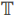, but this less common symbol is not rendered by all browsers, so I have stuck to T. Incidentally, the T stands for torus. This is because the Circle Group is topologically equivalent to a 1-torus. An exposition of Topology will have to wait for another book. However, the shape that most people would think of as being a torus (and which makes an appearance in Chapter 19), i.e. the surface of a quoit, is actually a 2-torus. A 2-torus is the direct product of two circles: 2-torus = S1 × S1 Higher dimensional toruses are the direct products of multiple circles: n-torus = S1 × S1 × … × S1 Where the product has n terms. Thus a 1-torus is the product of 1 circle, or simply a circle.  It will actually be identical for the case where n = 1, however we will need to consider n = 2 and n = 3 when we look at the Special Unitary Groups SU(2) and SU(3), so we need to develop the more general formulation here.  Or maybe more precisely rotating through the first angle followed by rotating through the second (which could be viewed as a form of addition).  A moment’s reflection will lead to the conclusion that is doesn’t matter in which order these steps are carried out. More explicitly for any square matrix M:Here H stands for Hermitian, which in turn stands for Charles Hermite, an esteemed 19th Century French Mathematician.  With reference to the last note, Physicists would tend to say such a matrix is Hermitian – there is a bit of a bun fight as to who “owns” Charles Hermite, the Mathematicians or the Physicists.
 Text: © Peter James Thomas 2016-17. Images: © Peter James Thomas 2016-17, unless stated otherwise. Published under a Creative Commons Attribution 4.0 International License.